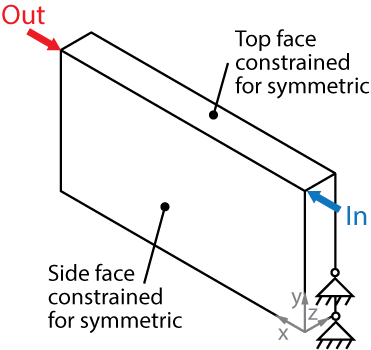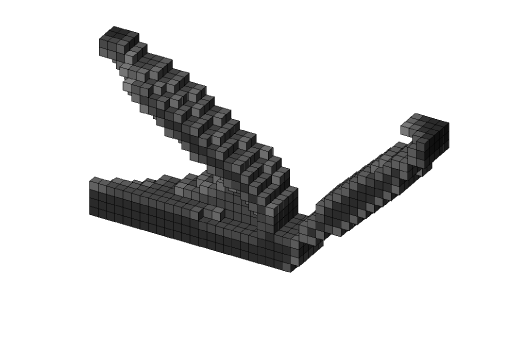# Compliant Mechanisms Synthesis – Top3d

In mechanical engineering, compliant mechanisms are flexible mechanisms that transfer an input force or displacement to another point through elastic body deformation. These are usually monolithic (single-piece) or jointless structures with certain advantages over the rigid-body, or jointed, mechanisms. In this tutorial, you will learn how to change the top3d program to solve compliant mechanism synthesis.

A compliant mechanism problem involves loading cases: input loading case and dummy loading case. The code also needs to implement a new objective function and its corresponding sensitivity analysis. To demonstrate this implementation, let us consider a three-dimensional force inverter problem as shown in figure below. With an input load defined in the positive direction, the design goal is to maximize the negative horizontal output displacement. Both the top face and the side force are imposed with symmetric constraints; i.e., nodes can only move within the plane.The new loading conditions as well as input and output points are defined as follows:

% USER-DEFINED LOAD DOFs
% [Input Dummy]
il = [0 nelx]; jl = [nely nely]; kl = [0 0];

F = sparse(loaddof,[1 2],[1 -1],ndof,2);


### Step.2: Define Boundary Conditions

The boundary conditions are defined as below:

% USER-DEFINED SUPPORT FIXED DOFs
% Top face
[iif,kf] = meshgrid(0:nelx,0:nelz);
fixednid = kf*(nelx+1)*(nely+1)+iif*(nely+1)+1;
fixeddof_t = 3*fixednid(:)-1;
% Side face
[iif,jf] = meshgrid(0:nelx,0:nely);
fixednid = iif*(nely+1)+(nely+1-jf);
fixeddof_s = 3*fixednid(:);
% Two pins
iif = [0 0]; jf = [0 1]; kf = [nelz nelz];
fixednid = kf*(nelx+1)*(nely+1)+iif*(nely+1)+(nely+1-jf);
fixeddof_p = [3*fixednid(:); 3*fixednid(:)-1; 3*fixednid(:)-2];
% Fixed DOFs
fixeddof = union(fixeddof_t,fixeddof_s);
fixeddof = union(fixeddof, fixeddof_p);
fixeddof = sort(fixeddof);

% Displacement vector
U = zeros(ndof,2);
freedofs = setdiff(1:ndof,fixeddof);


The external springs with stiffness $0.1$ are added at input and output points after line 71.

K(din,din) = K(din,din) + 0.1;
K(dout,dout) = K(dout,dout) + 0.1;</pre>


### Step.4: Changing objective function and sensitivity analysis

The expressions of the objective function and sensitivity are modified in lines 74-76.

U1 = U(:,1); U2 = U(:,2);
ce = reshape(sum((U1(edofMat)*KE).*U2(edofMat),2),[nely,nelx,nelz]);
c  = U(dout,1);
dc = penal*(E0-Emin)*xPhys.^(penal-1).*ce;


### Step.5: Changing OC subroutine

The convergence criteria for the bi-sectioning algorithm (lines 82-83) is improved by the following lines:

l1 = 0; l2 = 1e9; move = 0.1;
while (l2-l1)/(l1+l2) > 1e-4 && l2 > 1e-40


To improve the convergence stability, the damping factor of OC-method changes from 0.5 to 0.3 and also takes the positive sensitivities into account, then line 85 is changed to:

xnew = max(0,max(x-move,min(1,min(x+move,x.*(max(1e-10,-dc./dv/lmid)).^0.3))));


### Step.6: Running the programThe final design shown above is promoted by the line in the Matlab:

top3d(40,20,5,0.30,3.0,1.5)